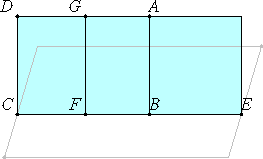# Proposition 18

If a straight line is at right angles to any plane, then all the planes through it are also at right angles to the same plane.

Let any straight line AB be at right angles to the plane of reference.

I say that all the planes through AB are also at right angles to the plane of reference.Let the plane DE be drawn through AB. Let CE be the intersection of the plane DE and the plane of reference.

I.11

Take a point F at random on CE, and draw FG from F at right angles to CE in the plane DE.

XI.Def.3

Now, since AB is at right angles to the plane of reference, therefore AB is also at right angles to all the straight lines which meet it and lie in the plane of reference, so that it is also at right angles to CE. Therefore the angle ABF is right.

I.28

But the angle GFB is also right, therefore AB is parallel to FG.

XI.8

But AB is at right angles to the plane of reference, therefore FG is also at right angles to the plane of reference.

XI.Def.4

Now a plane is at right angles to a plane when the straight lines drawn in one of the planes at right angles to the intersection of the planes are at right angles to the remaining plane. And FG, drawn in one of the planes DE at right angles to CE, the intersection of the planes, was proved to be at right angles to the plane of reference. Therefore the plane DE is at right angles to the plane of reference.

Similarly it can also be proved that all the planes through AB are at right angles to the plane of reference.

Therefore, if a straight line is at right angles to any plane, then all the planes through it are also at right angles to the same plane.

Q.E.D.

## Guide

This proposition is used in the proof of proposition XII.17.